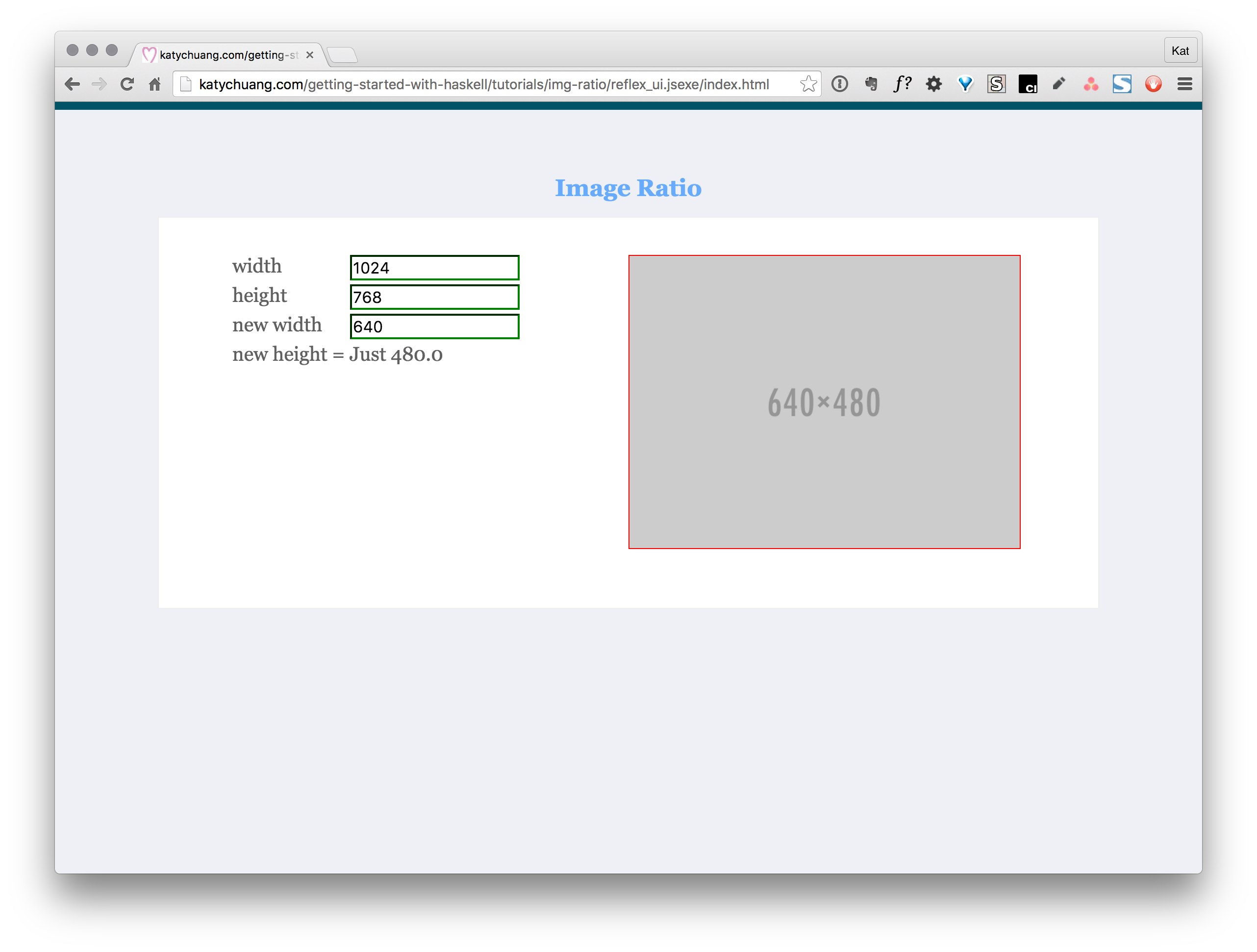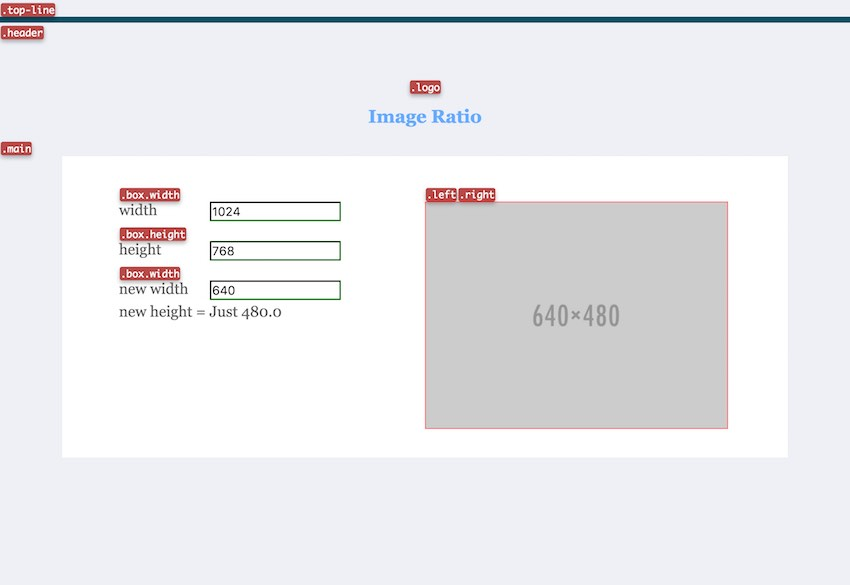# Working with multiple ReflexFRP text boxes

Filed under: programming

Last week during my flight to West Coast visit my parents for Chinese New Year, I spent sometime with my getting-started-with-haskell project.

Last Fall I started working on a web app version of the image ratio calculator, struggling to understand how the number inputs work with the calculations. Last spring/summer I was working calculating image rations (img-ratio.hs) to understand how Haskell functions could be defined and interacted with through ghci. Now I wanted a GUI interface.Reading through the calculator tutorial showed new functions I’ve never seen before. Luckily my brother gave me his wifi access code so I could look function definitions on hackage up during the flight.

Tip: Reading documentation is the first step to understanding new code; to find out how it is defined then you can better understand how it is used.

Here are some of the types that come from the Reflex-Dynamic module.

#### Dynamic Types…

``````mapDyn :: (Reflex t, MonadHold t m) => (a -> b) -> Dynamic t a -> m (Dynamic t b)

combineDyn :: forall t m a b c. (Reflex t, MonadHold t m) => (a -> b -> c) -> Dynamic t a -> Dynamic t b -> m (Dynamic t c)

constDyn :: Reflex t => a -> Dynamic t a

count :: (Reflex t, MonadHold t m, MonadFix m, Num b) => Event t a -> m (Dynamic t b)

dynText :: MonadWidget t m => Dynamic t String -> m ()
``````

I borrowed the numberInput function from the tutorial, added a string for plugging in the initial value.

``````numberInput :: (MonadWidget t m) => String -> m (Dynamic t (Maybe Double))
numberInput i = do
let errorState = Map.singleton "style" "border-color: red"
validState = Map.singleton "style" "border-color: green"
rec n <- textInput  def & textInputConfig_inputType .~ "number"
& textInputConfig_initialValue .~ i
& textInputConfig_attributes .~ attrs
result <- mapDyn readMay  _textInput_value n
attrs <- mapDyn (\r -> case r of
Just _ -> validState
Nothing -> errorState) result
return result
``````

I also created a function to create the label + text input combo:

``````box t i =
elClass "div" "box height"  do
el "label"  text t
y <- numberInput i
return y
``````

With both functions defined I could go ahead and write the first version of a working app. I hardcoded the initial values to get the 4:3 ratio as I wanted to focus on getting the reflex dom working. I’ll be going back in to improve the usability of the interface.

``````main = mainWidgetWithCss (embedFile "style.css")   do
elClass "div" "top-line"  return ()

elClass "h1" "logo"  text "Image Ratio"

elClass "div" "main"  do
elClass "div" "left"  do

x1 <- box "width" "1024"
y1 <- box "height" "768"

x2 <- box "new width" "640"
y2 <- box "new height" "480"

step1 <- combineDyn (\x y -> (*) <> x <*> y) x2 y1
step2 <- combineDyn (\x y -> (/) <> x <*> y) step1 x1

resultString <- mapDyn show step2  --(Dynamic Spider (Maybe Double))
text "new height = "
s <- dynText resultString
z <- box "new height" (show s) -- (Dynamic Spider String)
return ()

elClass "div" "right"  do
elAttr' "div" ("style" =: "width:400px; height:300px; border: 1px solid red; position: fixed;")  return ()
elAttr' "img" ("src" =: "http://placehold.it/640x480" <> "width" =: "400")  return ()

return ()
``````

This is how it turns out with the applied stylesheet:Files for this example:

Next I’m trying to work on displaying dynamic text in text boxes as soon as the user updates values. I think I’m going to have to take the value out of the monad. Here are some I found that work with monad types.

``````(=<<) :: Monad m => (a -> m b) -> m a -> m b
liftM :: Monad m => (a1 -> r) -> m a1 -> m r
fmap :: (a -> b) -> f a -> f b
``````